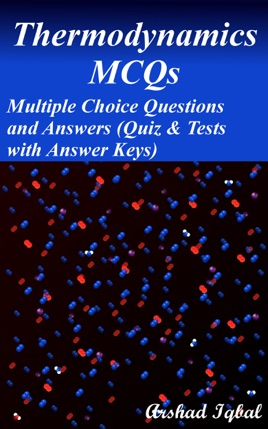• \$3.99

## Publisher Description

Thermodynamics multiple choice questions has 100 MCQs. Thermodynamics quiz questions and answers pdf, MCQs on applied thermodynamics, first law of thermodynamics MCQs with answers, second law of thermodynamics, reversible and irreversible processes and working fluid MCQs and quiz to practice exam prep tests.
Thermodynamics multiple choice quiz questions and answers pdf, thermodynamics exam revision and study guide with practice tests for online exam prep and interviews. Civil and mechanical engineering interview questions and answers to ask, to prepare and to study for jobs interviews and career MCQs with answer keys.
Applied thermodynamics quiz has 24 multiple choice questions. Reversible and irreversible processes quiz has 30 multiple choice questions. Second law of thermodynamics quiz has 20 multiple choice questions with answers. Working fluid quiz has 26 multiple choice questions.
Civil and mechanical engineering interview questions and answers pdf, MCQs on conservation of energy, entropy, energy, first law of thermodynamics, heat engine, heat work and system, irreversible processes, joule's law, liquid, vapors and gas, non-flow systems, perfect gas, polytrophic process, pressure, relationship between specific heat capacities, reversible non flow processes, specific enthalpies of perfect gas, specific heat capacity, state of working fluid, t-s diagram, temperature, the non-flow equation, the steady flow equation, units, thermodynamics worksheets for competitive exams preparation.